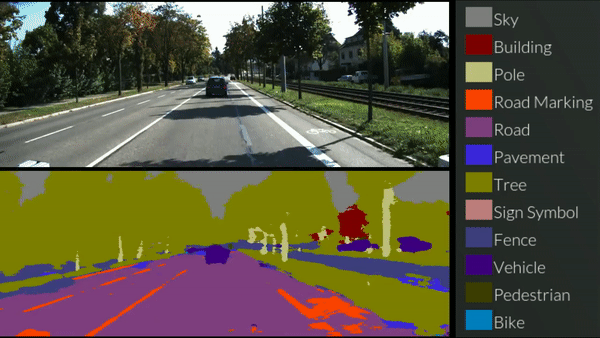## Similarities between Semantic Segmentation and Video Summarization

Compared with video summarization, semantic segmentation is a more widely studied topic in computer vision. Traditionally, video summarization and semantic segmentation are considered as two completely different problems in computer vision.The insight is that these two problems in fact share a lot of similarities.

• In semantic segmentation, the input is a 2D image with 3 color channels (RGB). The output of semantic segmentation is a 2D matrix with the same spatial dimension as the input image, where each cell of the 2D matrix indicates the semantic label of the corresponding pixel in the image.
• In video summarization, let us assume that each frame is represented as a K-dimensional vector. This can be a vector of raw pixel values or a pre-computed feature vector. Then the input to video summarization is a 1D image (over temporal dimension) with K channels. The output is a 1D matrix with the same length as the input video, where each element indicates whether the corresponding frame is selected for the summary.

Conclusion: Although semantic segmentation and video summarization are two different problems, they only differ in terms of the dimensions of the input (2D vs. 1D) and the number of channels (3 vs. K).

Hence, by establishing the connection between these two tasks, we can directly exploit models in semantic segmentation and adapt them for video summarization.